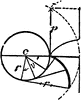# Construction Of An Evolute Of A CircleAn illustration showing how to construct an evolute of a circle. “Given the pitch p, the angle v, and radius r. Divide the angle v into a number of equal parts, draw the radii and tangents for each part, divide the pitch p into an equal number of equal parts, then the first tangent will be one part, second two parts, third three parts, etc., and so the Evolute is traced.”

### Galleries

Analytical Geometry

### Source

Albert A. Hopkins Scientific America: Handy Book Of Facts And Formulas (New York: Munn & Co., Inc., 1918) 39

TIFF (full resolution)

1948×2400, 269.3 KiB

Large GIF

831×1024, 40.4 KiB

Medium GIF

519×640, 23.4 KiB

Small GIF

259×320, 10.2 KiB